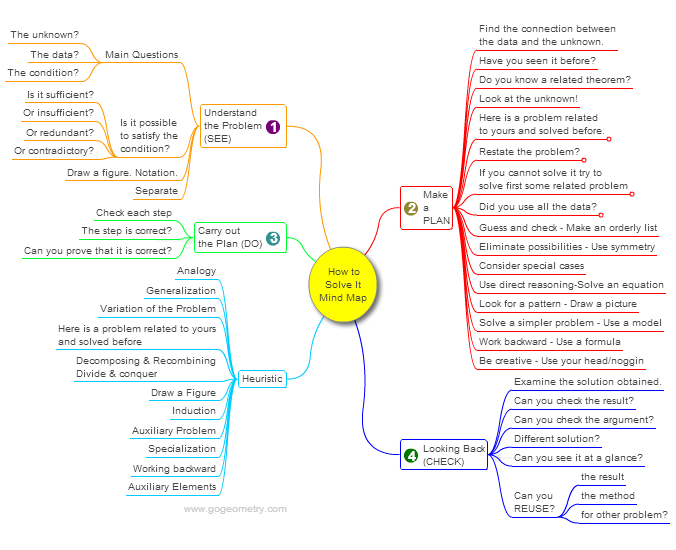# Geometry Problem 1037: Cracking the Inradius Code: Unveiling the Relationship of Triangles and their Inradii for School and College Level.

< PREVIOUS PROBLEM  |  NEXT PROBLEM >

The figure below depicts triangle ABC, with points A1, B1, and C1 located on BC, AC, and AB, respectively. These points are chosen such that the inradii of triangles AB1C1, BA1C1, and CA1B1 are all equal to d. Let r represent the inradius of triangle ABC, and r1 denote the inradius of triangle A1B1C1. Prove that r1 = r - d.## Mind Map: George Pólya's 1945 book, "How to Solve It"

George Pólya's 1945 book "How to Solve It, A new aspect of Mathematical Method", is a book describing methods of problem solving. It suggests the following steps when solving a mathematical problem: (1) First, you have to understand the problem. (2) After understanding, then make a plan. (3) Carry out the plan. (4) Look back on your work. How could it be better?
A mind map is a diagram used to visually organize information, knowledge, concepts or ideas.Home | SearchGeometry | Problems | All Problems | Open Problems | Visual Index | 10 Problems | Problems Art GalleryArt | 1031-1040 | Triangle | Circle | Incircle | Areas | Triangle area | Perimeter | Tangent Line | Email | by Antonio Gutierrez

Add a solution to the problem 1037
Last updated: Sep 19, 2014